# Latest word problems

1. Trees avgThe worker planted 96 trees on Monday, 120 on Tuesday and 61 trees on Wednesday. How many trees did he plant on Thursday if he averaged 105 trees per day?
2. Inscribed circle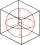A circle is inscribed at the bottom wall of the cube with an edge (a = 1). What is the radius of the spherical surface that contains this circle and one of the vertex of the top cube base?
3. 1st drug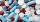First drug pack has an active ingredient ratio of L1: L2 = 2: 1 Second drug pack have a ratio of active ingredients L1: L2 = 1: 3 In which ratio do we have to mix the two packages so that the ratio of substances L1: L2 = 1: 2?
4. Two integers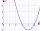Two integers, a and b, have a product of 36. What is the least possible sum of a and b?
5. Construct rhombusConstruct rhombus ABCD if given diagonal length | AC | = 8cm, inscribed circle radius r = 1.5cm
6. A number 2A number decreased by the difference between four and the number
7. Rectangular gardenThe perimeter of Peter's rectangular garden is 98 meters. The width of the garden is 60% shorter than its length. Find the dimensions of the rectangular garden in meters. Find the garden area in square meters.
8. The taxThe tax per gallon of gasoline in California is \$0.477. If you fill your gasoline tank with 14.4 gallons of gasoline in California, how much will you pay in taxes? Round to the nearest cent.
9. Blueberries collecting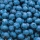Marie collects blueberries 2 l in 1 hour. Began collecting at 8.00 pm, Joe collects 1.5 l in 1 hour. Peter 1 l for 1 hr. And they began to gather at 08.30 pm. Together they collect 10 l. At what time will they all have collected?
10. Two portsFrom port A on the river, the steamer started at an average speed of 12 km/h towards port B. Two hours later, another steamer departed from A at an average speed of 20 km/h. Both ships arrived in B at the same time. What is the distance between ports A an
11. Apple varieteThis year, my uncle harvested 350 kg of apples, including 105 kilograms of Ontario, and the rest is Jonathan. Determine what percentage of the Jonathan apples.
12. Art schoolEvery fifth pupil 9A goes to art school. How many percent of pupils in class 9A go to art school?
13. Eight masonsEight masons will plaster a wall with an area of 1440 m2 in 9 days. They work 8 hours a day. How much area will plaster 6 masons in 4 hours?
14. Rectangle fieldThe field has a shape of a rectangle having a length of 119 m and a width of 19 m. , How many meters have to shorten its length and increase its width to maintain its area and circumference increased by 24 m?
15. Three parallelsThe vertices of an equilateral triangle lie on 3 different parallel lines. The middle line is 5 m and 3 m distant from the end lines. Calculate the height of this triangle.
16. An animal shelter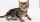An animal shelter spends \$5.50 per day to care for each bird and \$8.50 per day to care for each cat. Nicole noticed that the shelter spent \$283.00 caring for birds and cats on Wednesday. Nicole found a record showing that there were a total of 40 birds an
17. Where and when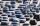The truck left Kremnica at 11:00 h at a speed of 60km/h. At 12:30 h, the passenger car started at an average speed of 80km/h. How many kilometers from Kremnica will the passenger car reach truck, and when?
18. Land boundaryThe land has the shape of a right triangle. The hypotenuse has a length of 30m. The circumference of the land is 72 meters. What is the length of the remaining sides of the land boundary?
19. Ice skates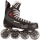Ice skates were raised twice, the first time by 25%, the second time by 10%. After the second price, their cost was 82.5 euros. What was the original price of skates?
20. Parallels and one secant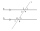There are two different parallel lines a, b and a line c that intersect the two parallel lines. Draw a circle that touches all lines at the same time.

Do you have an interesting mathematical word problem that you can't solve it? Submit math problem, and we can try to solve it.

We will send a solution to your e-mail address. Solved examples are also published here. Please enter the e-mail correctly and check whether you don't have a full mailbox.

Please do not submit problems from current active competitions such as Mathematical Olympiad, correspondence seminars etc...